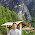Tuesday, July 30, 2013

When is 1 + 1 = 3?

It has always been heard, Is 1+1=3? There are many views on it. One popular view is 1+1 is 2 but the capital gain from the transaction is around 1 which adds to 2 and results as 3. Which means that 1 + 1 and profit of 1 makes it to three. One view is also that a man and a woman marries and  produces a kid and is then called 1+1=3 the third one as the baby born to them. There are many other views and I have my view which  I will prove mathematically later on.

My view is simple, I will use only mathematical tools to prove it.
1 + 1 = 3
Though you may find this as an equation, it may be an equation but I say it is an infinite equation which currently I understand some when I have to deal with 4 minimal decimal points. A number in itself is infinite.

1 and 1.0000000000000000001 is same mathematically. It is called the decimal point which is denoted by a dot “.” And there is a rule in mathematics that less than 5 is rounded down and more than 5 is rounded up to the next nearest number.

Now I will prove with the following equation;

1.4 (which is generally said as 1 if rounded down, in these case anything lesser than 1.5 and more than 1.25 will be applicable here)

Which is 1.4 + 1.4 = 2.8

Since 1.4 rounded down is 1 and 2.8 which rounded up is 3, we can say it is

1 + 1 = 3

Thus proved that under circumstances that 1 has decimal points and sums to more than 2.5 will result to 3 as rounding up factor applies.

1 comment:

1.Brother don;t try to mess with the mathematical equation but most reason you ve provided makes some sense.